Next: Antenna Arrays Up: Radiation and Scattering Previous: Basic Antenna Theory

# Antenna Directivity and Effective Area

We have seen that standard antennas emit more radiation in some directions than in others. Indeed, it is topologically impossible for an antenna to emit transverse waves uniformly in all directions (for the same reason that it is impossible to comb the hair on a sphere in such a manner that there is no parting). One of the aims of antenna engineering is to design antennas that transmit most of their radiation in a particular direction. By a reciprocity argument, such an antenna, when used as a receiver, is preferentially sensitive to radiation incident from the same direction.

The directivity or gain of an antenna is defined as the ratio of the maximum value of the power radiated per unit solid angle to the average power radiated per unit solid angle: that is,(1242)

Thus, the directivity measures how much more intensely the antenna radiates in its preferred direction than a mythical isotropic radiator'' would when fed with the same total power. For a Hertzian dipole, the gain is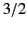. For a half-wave antenna, the gain is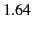. To achieve a directivity that is significantly greater than unity, the antenna size needs to be much larger than the wavelength. This is usually achieved using a phased array of half-wave, or full-wave, antennas.

Antennas can be used to receive, as well as emit, electromagnetic radiation. The incoming wave induces a voltage that can be detected in an electrical circuit connected to the antenna. In fact, this process is equivalent to the emission of electromagnetic waves by the antenna viewed in reverse. In the theory of electrical circuits, a receiving antenna is represented as an emf connected in series with a resistor. The emf,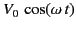, represents the voltage induced in the antenna by the incoming wave. The resistor,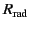, represents the power re-radiated by the antenna (here, the real resistance of the antenna is neglected). Let us represent the detector circuit as a single load resistor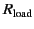connected in series with the antenna. How can we choosesuch that the maximum power is extracted from the incoming wave and transmitted to the load resistor? According to Ohm's law,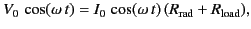(1243)

where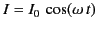is the current induced in the circuit. The power input to the circuit is(1244)

The power transferred to the load is(1245)

Finally, the power re-radiated by the antenna is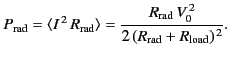(1246)

Note that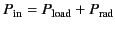. The maximum power transfer to the load occurs when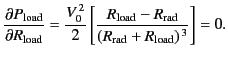(1247)

Thus, the maximum transfer rate corresponds to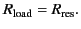(1248)

In other words, the resistance of the load circuit must match the radiation resistance of the antenna. For this optimum case,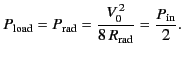(1249)

So, even in the optimum case, half of the power absorbed by the antenna is immediately re-radiated. If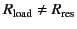then more than half of the absorbed power is re-radiated. Clearly, an antenna that is receiving electromagnetic radiation is also emitting it. This is how the BBC (allegedly) catch people who do not pay their television license fee in the UK. They have vans that can detect the radiation emitted by a TV aerial while it is in use (they can even tell which channel you are watching!).

For a Hertzian dipole antenna interacting with an incoming wave whose electric field has an amplitude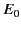, we expect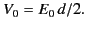(1250)

Here, we have used the fact that the wavelength of the radiation is much longer than the length of the antenna, and that the relevant emf develops between the two ends and the centre of the antenna. We have also assumed that the antenna is properly aligned (i.e., the radiation is incident perpendicular to the axis of the antenna). The Poynting flux of the incoming wave is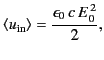(1251)

whereas the power transferred to a properly matched detector circuit is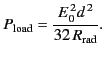(1252)

Consider an idealized antenna in which all incoming radiation incident on some area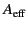is absorbed, and then magically transferred to the detector circuit with no re-radiation. Suppose that the power absorbed from the idealized antenna matches that absorbed from the real antenna. This implies that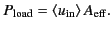(1253)

The quantity, which is called the effective area of an antenna, is the area of the idealized antenna that absorbs as much net power from the incoming wave as the actual antenna. Alternatively,is the area of the incoming wavefront that is captured by the receiving antenna and fed to its load circuit. Thus,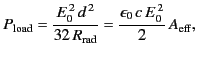(1254)

giving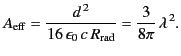(1255)

It is clear that the effective area of a Hertzian dipole antenna is of order the wavelength squared of the incoming radiation.

We can generalize from this analysis of a special case. The directivity of a Hertzian dipole is. Thus, the effective area of the isotropic radiator (the mythical reference antenna against which directivities are measured) is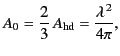(1256)

or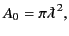(1257)

where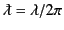. Here, we have used the formal definition of the effective area of an antenna:is that area which, when multiplied by the time-averaged Poynting flux of the incoming wave, equals the maximum power received by the antenna (when its orientation is optimal). Clearly, the effective area of an isotropic radiator is the same as the area of a circle whose radius is the reduced wavelength,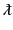.

We can take yet one more step, and conclude that the effective area of any antenna of directivity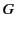is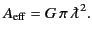(1258)

Of course, to realize this full capture area, the antenna must be orientated properly.

Let us calculated the coupling, or insertion loss, of an antenna-to-antenna communications link. Suppose that a generator delivers the power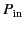to a transmitting antenna, which is aimed at a receiving antenna a distance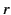away. The (properly aligned) receiving antenna then captures and delivers the power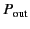to its load circuit. From the definition of directivity, the transmitting antenna produces the time-averaged Poynting flux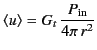(1259)

at the receiving antenna. The received power is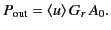(1260)

Here,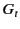is the gain of the transmitting antenna, and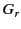is the gain of the receiving antenna. Thus,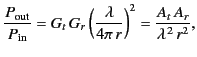(1261)

where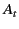and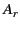are the effective areas of the transmitting and receiving antennas, respectively. This result is known as the Friis transmission formula. Note that the insertion loss depends on the product of the gains of the two antennas. Thus, a properly aligned communications link has the same insertion loss operating in either direction.

A thin wire linear antenna might appear to be essentially one-dimensional. However, the concept of an effective area shows that it possesses a second dimension determined by the wavelength. For instance, for a half-wave antenna, the gain of which is, the effective area is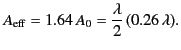(1262)

Thus, we can visualize the capture area as a rectangle that is the physical length of the antenna in one direction, and approximately one quarter of the wavelength in the other.Next: Antenna Arrays Up: Radiation and Scattering Previous: Basic Antenna Theory
Richard Fitzpatrick 2014-06-27